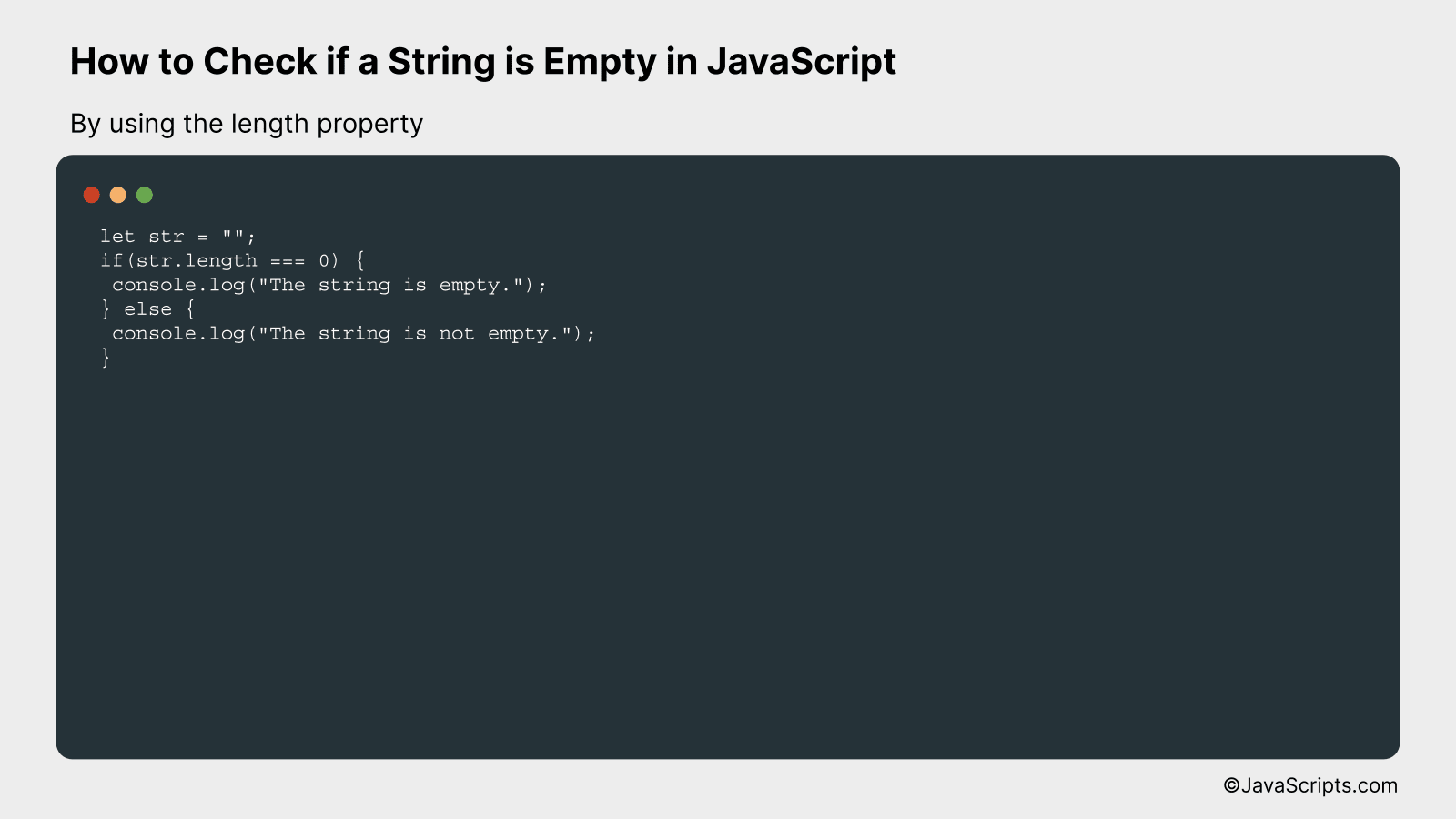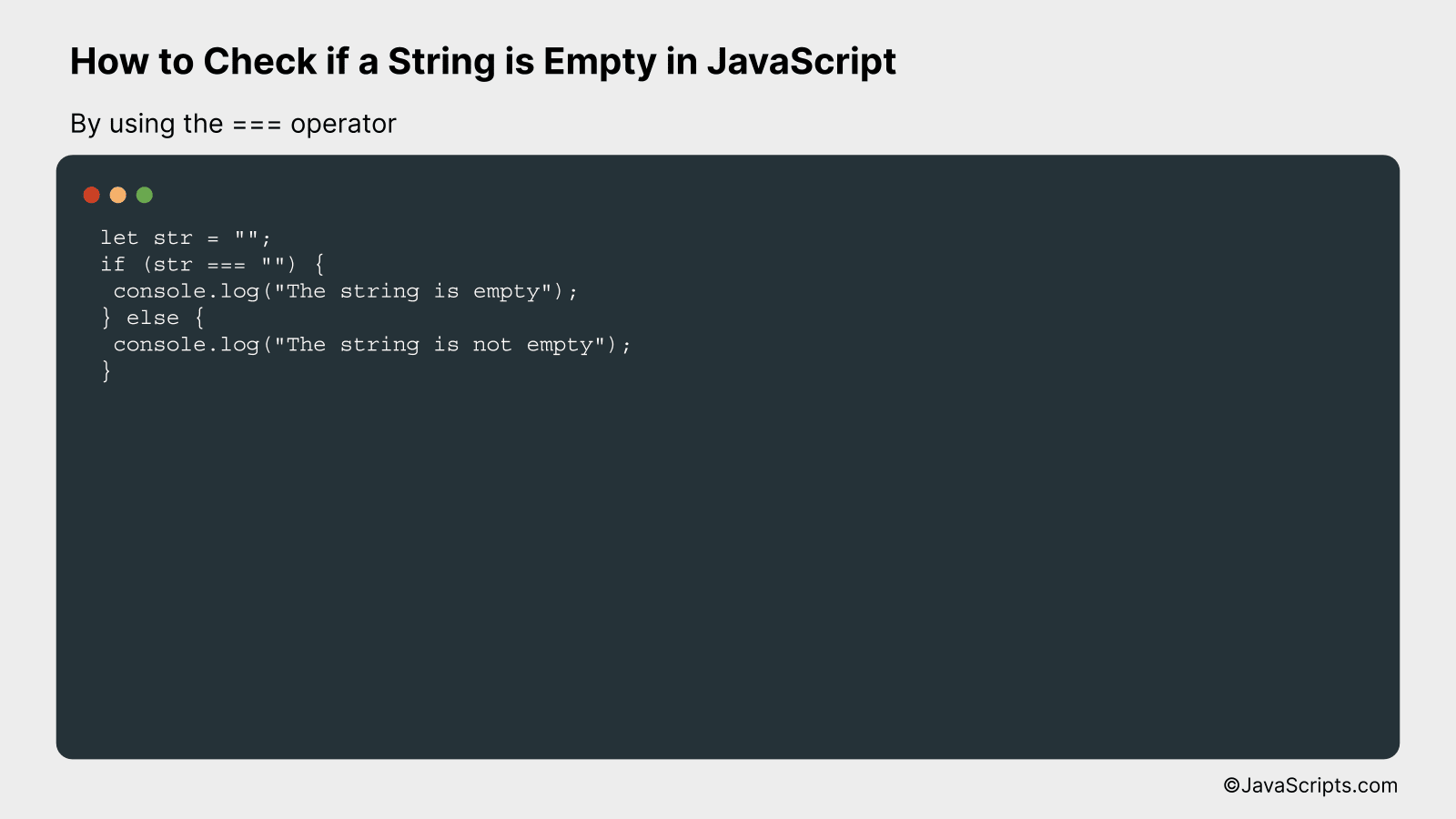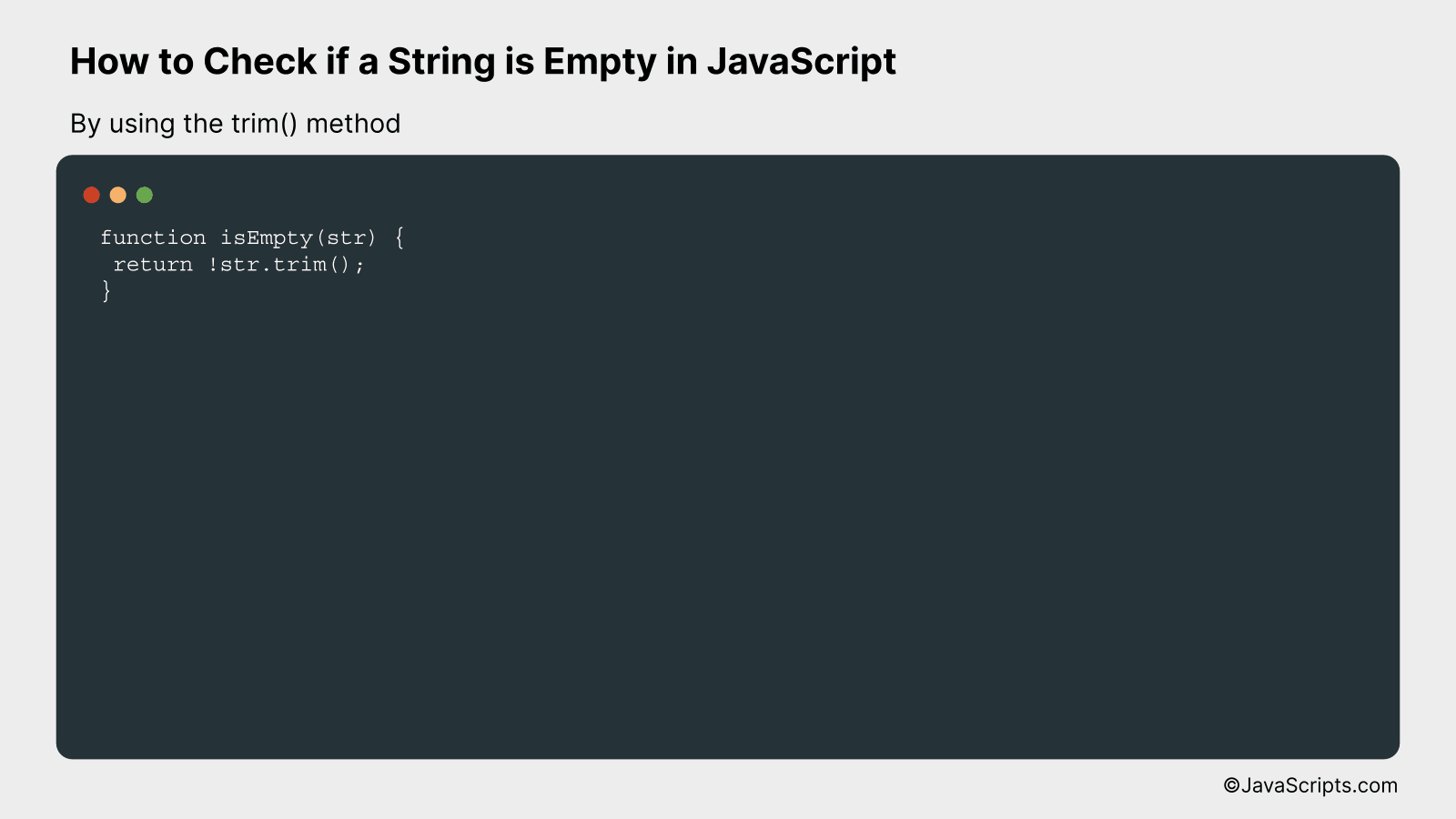# How to Check if a String is Empty in JavaScript

Web development is an exciting field, and JavaScript is one of the key tools in a developer’s kit. Often, there arises a need to verify if a string is empty. Let’s dive into how we can achieve this using JavaScript.

You’ll find this guide handy, whether you’re a seasoned coder or just starting out. We’ll walk together through a simple, step-by-step process. Remember, understanding these fundamentals is crucial to building a robust JavaScript application.

In this post, we will be looking at the following 3 ways to check if a string is empty in JavaScript:

• By using the length property
• By using the === operator
• By using the trim() method

Let’s explore each…

## #1 – By using the length propertyThe length property in JavaScript is used to determine the number of characters in a string. To check if a string is empty, we can check if the length of the string is zero. If the length is zero, then the string is empty. For example, if we have a string, str, we can check if it’s empty by using the code str.length === 0.

``````
let str = "";
if(str.length === 0) {
console.log("The string is empty.");
} else {
console.log("The string is not empty.");
}
``````

### How it works

In JavaScript, the .length property returns the number of characters in a string. By using the === operator, we can check if the length of the string is exactly zero, which implies that the string is empty.

• The variable ‘str’ is initialized with an empty string.
• The ‘if’ statement is used to check the condition. The condition here is str.length === 0. This will return true if the length of the string is zero and false if it’s not.
• If the condition returns true (i.e., the string length is zero), the code within the ‘if’ block will be executed. In our case, it will log “The string is empty” to the console.
• If the condition returns false (i.e., the string length is not zero), the code within the ‘else’ block will be executed. In our case, it will log “The string is not empty” to the console.

## #2 – By using the === operatorThe concept is pretty straightforward: we are going to use the strict equality operator (===) in JavaScript to check if a string is empty. The strict equality operator compares both the value and the type of the operands, making sure they are strictly equal. Hence, if the string is empty, it will return true, otherwise, it will return false.

``````
let str = "";
if (str === "") {
console.log("The string is empty");
} else {
console.log("The string is not empty");
}
``````

### How it works

The strict equality operator ensures a precise comparison. In this case, it checks whether the value and type of the ‘str’ string are strictly equal to an empty string. If the ‘str’ string is empty, the condition inside the ‘if’ statement becomes true, resulting in a console log message of “The string is empty”. If the ‘str’ string is not empty, the ‘else’ block executes, logging “The string is not empty”.

• Firstly, we initialize a variable ‘str’ with the string that we want to check.
• We then setup an ‘if’ condition to check if the ‘str’ variable is strictly equal to an empty string.
• If the condition is true, meaning ‘str’ is an empty string, we print out “The string is empty” to the console.
• If the condition is false, meaning ‘str’ is not an empty string, we proceed to the ‘else’ block and print out “The string is not empty” to the console.
• The reason we use the strict equality operator (===) instead of the equality operator (==) is to avoid type coercion, which could lead to unexpected results. The strict equality operator checks both the value and the type, ensuring a more precise comparison.

## #3 – By using the trim() methodThe JavaScript `trim()` method is used to remove white spaces from both ends of a string. To check if a string is empty, you can use this method to remove any possible white spaces and then check if the result is an empty string. This way, even if a string contains only spaces, it will still be considered empty.

For example, if we have a string

let str = ” “;

Using `trim()` method, it would be considered as an empty string.

``````
function isEmpty(str) {
return !str.trim();
}
```
```

### How it works

This function checks if a string is empty or only contains white spaces. It uses the JavaScript `trim()` method to remove any white spaces from both ends of the string and then checks if the resulting string is empty.

• The function `isEmpty` is defined with one parameter `str`, which is the string to check.
• The `trim()` method is called on the string `str`. This removes any white spaces from both ends of the string.
• The `!` operator is used to invert the Boolean value of the string. If the string is empty, it will return `false`, and the `!` operator will invert this to `true`. If the string is not empty, it will return `true`, and the `!` operator will invert this to `false`.
• So, if the string is empty or only contains white spaces, the function will return `true`. Otherwise, it will return `false`.

Related:

In conclusion, checking if a string is empty in JavaScript is an essential skill in coding. It’s as simple as using an equality operator to compare your string with an empty string or by using the length property of a string.

Remember, an empty string can be due to undefined, null, or empty values. So always consider these cases when writing your code. Happy coding!